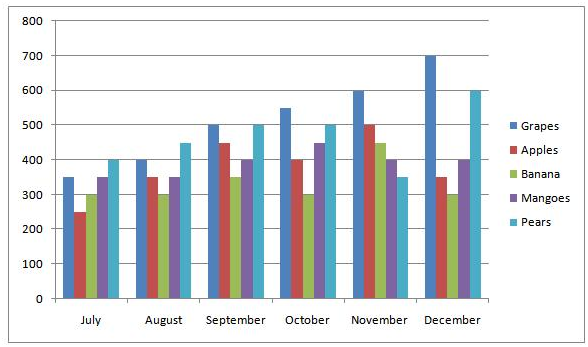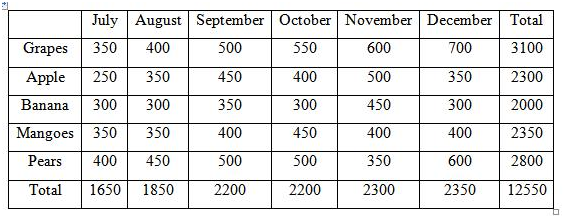# SBI Clerk Prelims 2021 Quantitative Aptitude Questions (Day-59)

Dear Aspirants, Our IBPS Guide team is providing new series of Quantitative Aptitude Questions for SBI Clerk Prelims 2020 so the aspirants can practice it on a daily basis. These questions are framed by our skilled experts after understanding your needs thoroughly. Aspirants can practice these new series questions daily to familiarize with the exact exam pattern and make your preparation effective.

Start Quiz

Wrong number series

Direction (1-5): Find the wrong term in the series given below.

1) 51, 60, 79, 134, 215, 336

A.51

B.79

C.215

D.60

E.134

2) 151, 182, 251, 386, 605, 956

A.151

B.182

C.251

D.605

E.386

3) 2, 3, 60, 420, 3360, 30240

A.30240

B.60

C.420

D.3

E.3360

4) 24, 24, 33, 39, 51, 57

A.51

B.24

C.57

D.39

E.33

5) 38, 52, 94, 164, 268, 388

A.94

B.38

C.52

D.164

E.268

Bar graph

Direction (6-10): Study the following information carefully and answer the questions.

The bar graph shows the exports of 5 different fruits over a 6 month period.6) How many kilogram of fruits were exported of all 5 varieties from September to November?

A.6250

B.6400

C.6500

D.6700

E.6850

7) What is the percentage increase in fruits exported from July to December for Grapes and Apples taken together?

A.80%

B.45%

C.75%

D.70%

E.65%

8) What is the difference between the exports in July and October of all 5 fruit varieties?

A.100

B.650

C.400

D.550

E.700

9) What percentage of Bananas from September was exported over the export of the same fruit in 6 month period?

A.12.5%

B.17.5%

C.14.5%

D.13.6%

E.16.5%

10) If January compared to December saw 30% growth in fruits exported of Grapes and Bananas and also if it saw 40% decrease of other fruits, then what will be the export of all 5 varieties in January?

A.2860

B.2530

C.2250

D.2220

E.2110

51+ 32 = 60

60 + 52 = 85

85 + 72 = 134

134 + 92= 215

215 + 112 = 336

151 + 33+ 4 = 182

182 + 43+ 5 = 251

251 +53+ 6 = 382

382 +63+ 7 = 605

605 + 73+ 8 = 956

The pattern is as follows:

2×5=10

10 x 6 = 60

60 x 7 = 420

420 x 8 = 3360

3360 x 9 = 30240

The next number is obtained by adding sum of digits of the previous number

24 + 2 + 4 = 30

30 + 3 + 0 = 33

33 + 3 + 3 = 39

39 + 3 + 9 = 51

51 + 5 + 1 = 57

The pattern is as follows:

38 + 14 * 1 = 52

52 + 14 * 3 = 94

94 + 14 * 5 = 164

164 + 14 * 7 = 262

262 + 14* 9 = 388

Directions (6-10) :Required value = 2200 + 2200 + 2300 = 6700 kg

July = 350 + 250 = 600

December = 700 + 350 = 1050

Percentage Increase = 100 *[(1050-600)/600]= 75%

Difference = 2200 – 1650 = 550

Total number of Bananas = 2000

Required percentage = 100*350/2000 = 17.5%